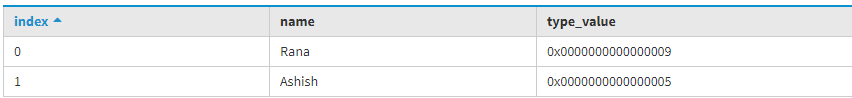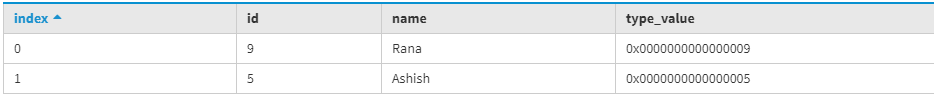# Blob conversion function in Cassandra

• Last Updated : 03 Dec, 2019

In Cassandra, Blob data type represents constant hexadecimal data type and the number is defined as 0[xX](hex)+ where hex means hexadecimal. It is useful to store a short string or small images.

In this article we will discuss Blob conversion function which converts the native types into binary data (blob):

```typeAsBlob(value)
blobAsType(value) ```

Let’s understand with an example.

To create table function_test1 used the following CQL query.

```CREATE TABLE function_test1 (
Name varchar PRIMARY KEY,
type_value blob
); ```

To insert data into the table using the following CQL query.

```INSERT INTO function_test1 (Name, type_value)
VALUES ('Ashish', bigintAsBlob(5));
INSERT INTO function_test1 (Name, type_value)
VALUES ('Rana', bigintAsBlob(9));  ```

To read the data using the following CQL query.

```SELECT *
FROM function_test1; ```

Output:Now, to convert blobAsBigint then first update the table by using the following CQL query.

`ALTER TABLE function_test1 ADD Id bigint; `

Now, again insert data into the updated table. So, let’s have a look.

```INSERT INTO function_test1 (Name, Id)
VALUES ('Ashish', blobAsBigint(0x0000000000000005));
INSERT INTO function_test1 (Name, Id)
VALUES ('Rana', blobAsBigint(0x0000000000000009)); ```

To see the final output used the following CQL query.

```SELECT *
FROM function_test1; ```

Output:My Personal Notes arrow_drop_up